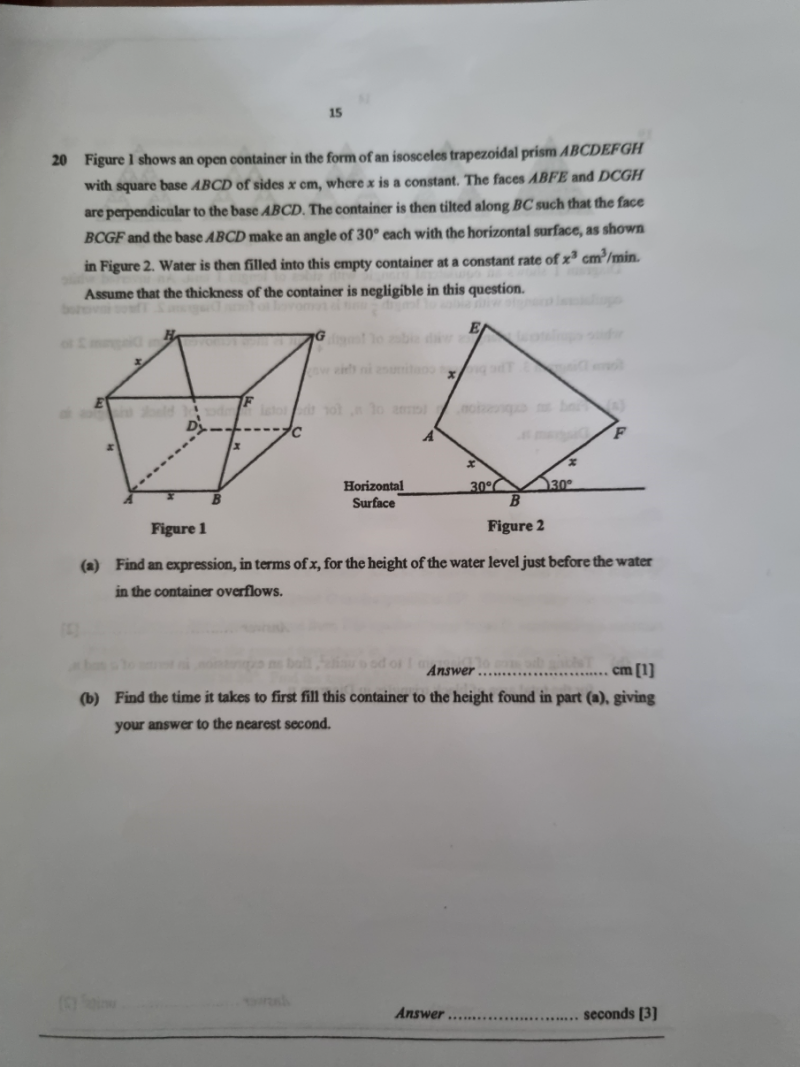# QuestionThank you

(a)  Let the point on the ground below point A be O

so AO is the height of the water level before it overflows.

AO/x = sin 30

AO = (1/2)x cm

Hence height of water level just before it overflows is (1/2) x  cm

(b)  Triangle AFB is the base area of the water volume and height is x

AF/x = 2 cos 30 = 2 (√3/2) = √3

So AF = (√3)x

Area of AFB = (1/2)(√3 (x))(1/2)x =  (√3/4) x2

Volume of water = (√3/4) x (x)  = (√3/4) x3

x3 —-> 60s

(√3/4) x —–> 60 x (√3/4)   = 25.98 ~ 26 seconds to the nearest seconds.

Hence time taken to fill the water to the height in (a) is ~ 26 seconds.

0 Replies 1 Like ✔Accepted Answer

(a) Make point O the point on the ground beneath point A.

Consequently, AO represents the height of the water before it overflows.

A = 30 sin AO/x

AO = (1/2)x cm

The water level is therefore (1/2) x cm high immediately before it overflows. basketball stars

1 Reply 1 Like

That’s fast!
Thank you very much, Ivory Morissette. word wipe

0 Replies 0 Likes